# Data

Do you know a bat always takes a left turn while leaving a cave. This was just an example of a type of data that I shared with you. Data can be of several types: data that you input into a computer program to get an output; it can also be statistics of a country's population. In fact, this information that you are reading at the moment can also be classified as data.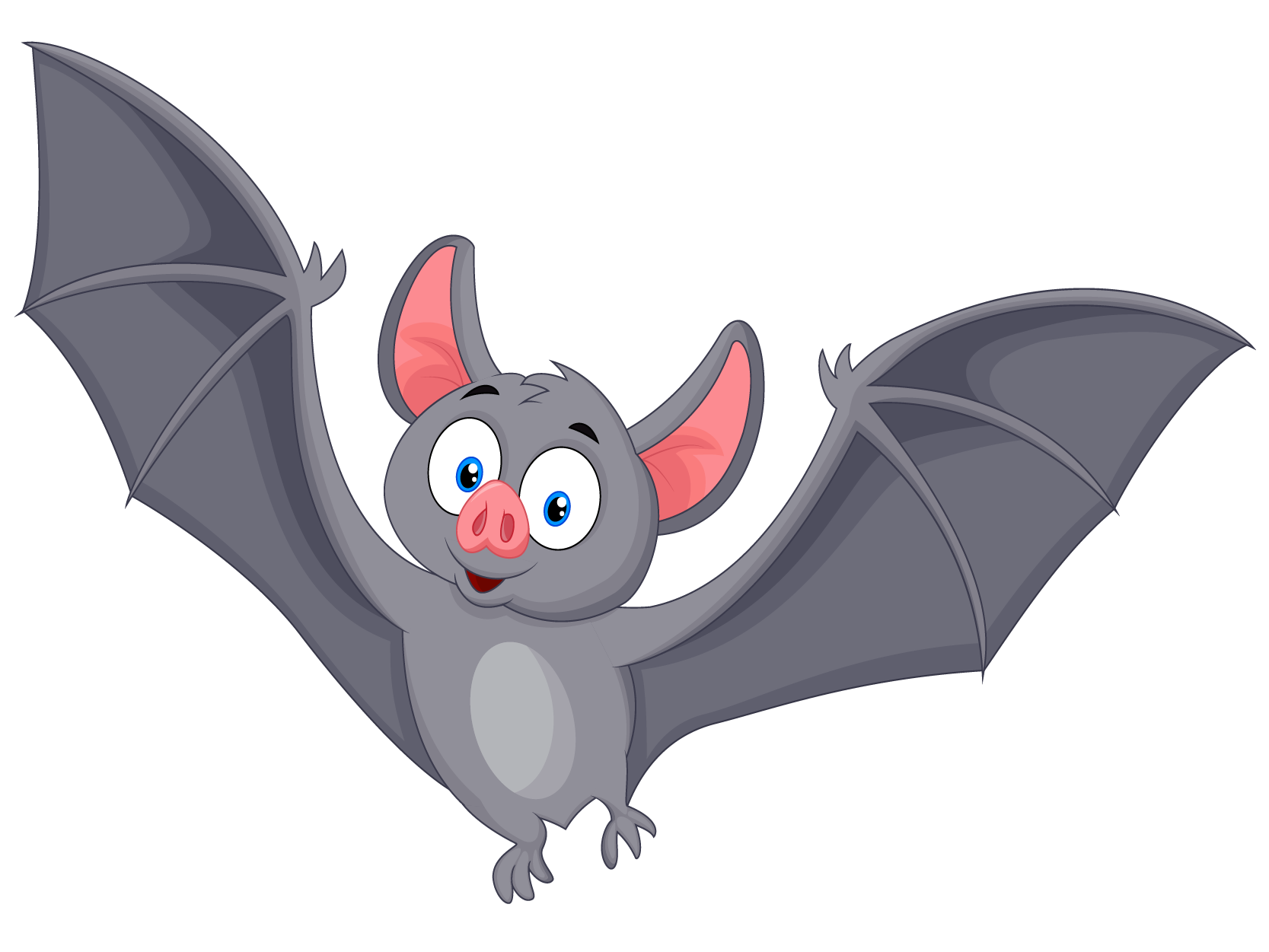Once you get your fundamentals in place, you will be acquainted with the various types of data, and even be tempted to solve our data examples at the end of the chapter.

## Lesson Plan

 1 What is Meant by Data? 2 Important Notes on Data 3 Solved Examples on Data 4 Challenging Questions on Data 5 Interactive Questions on Data

## What is Meant by Data?

Data in math can be any information or fact. It can be a number, an observation, an alphabet, or any collective knowledge about anything.

### Example:

• A set of numbers such as $${1, 2, 3, 4, 5}$$
• The list of student names in a class
• Physical attributes such as age, height, weight, etc.

Data handling refers to the process of gathering, recording, and presenting information in a way that is helpful to others. Data is usually represented in the form of pictographs, bar graphs, pie charts, histograms, line graphs, etc.

Below given are the images that show different ways to represent data:

### Pictograph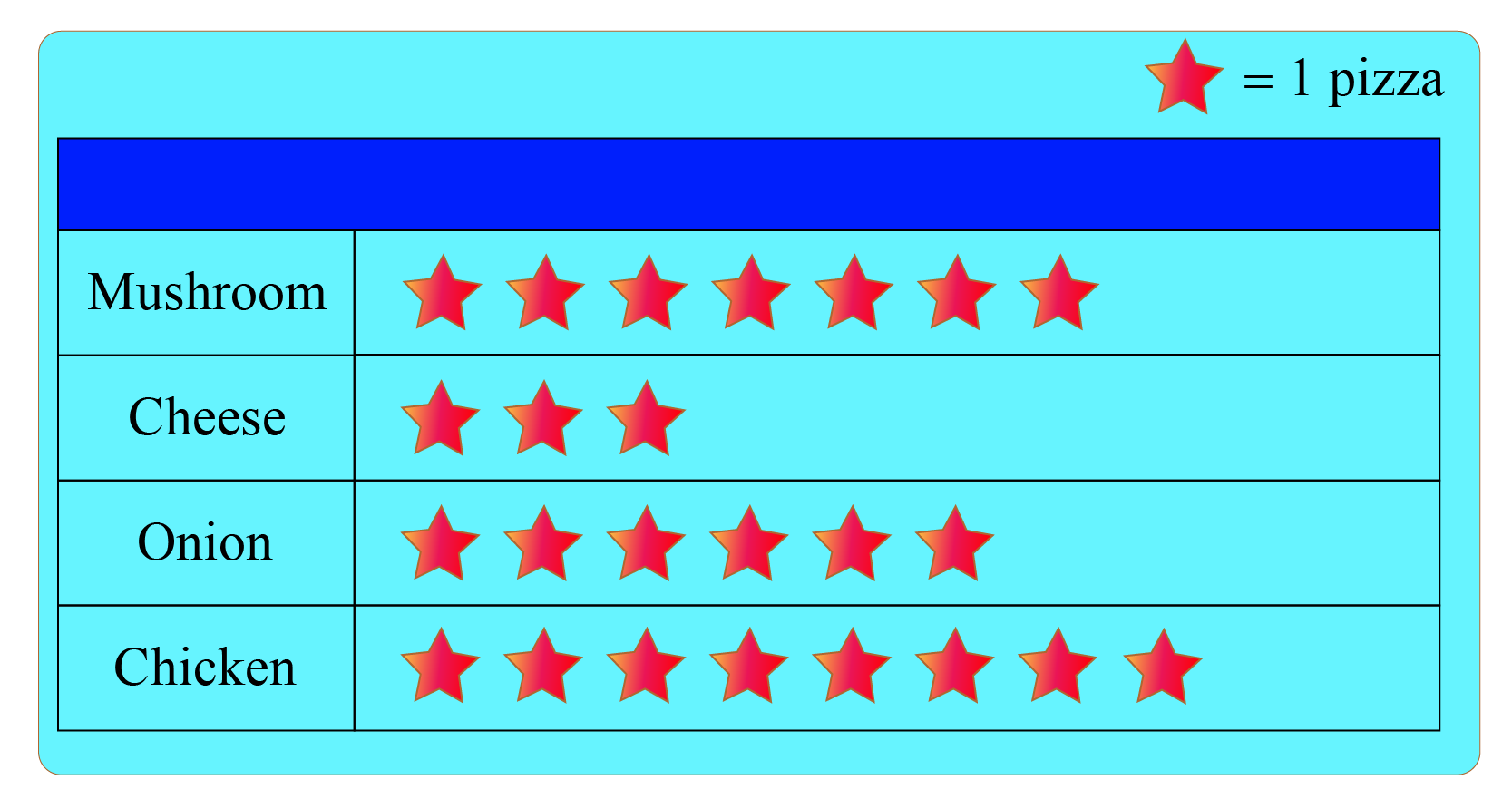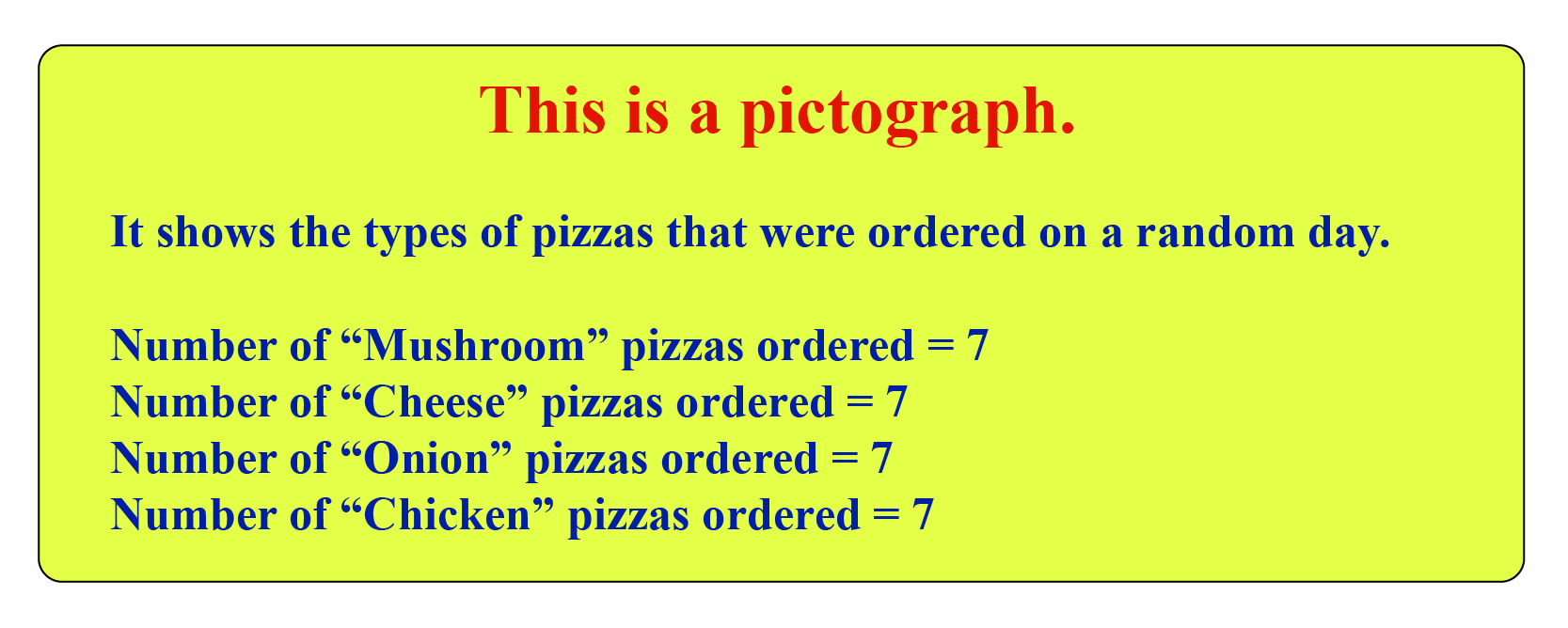### Pie-Chart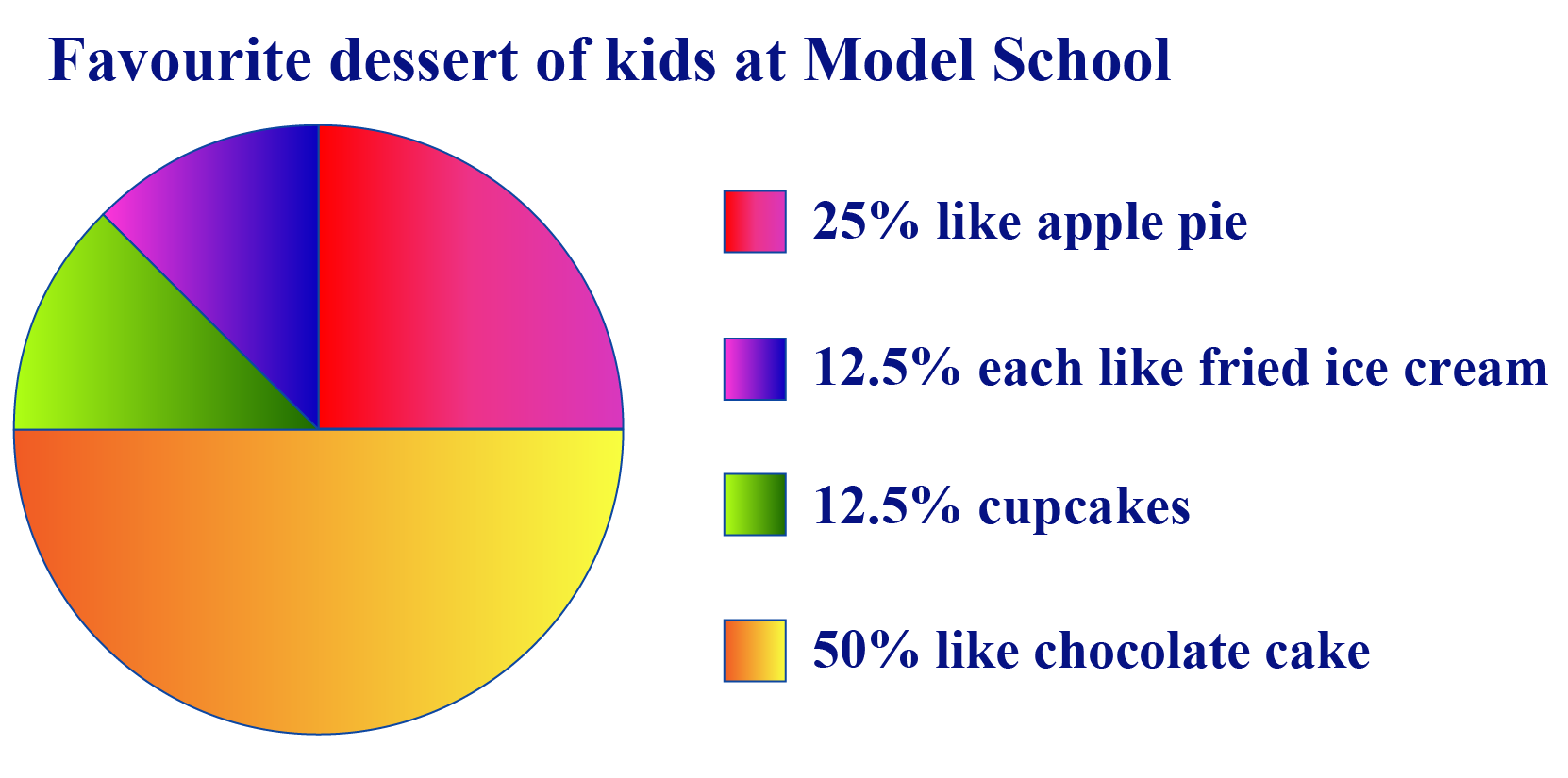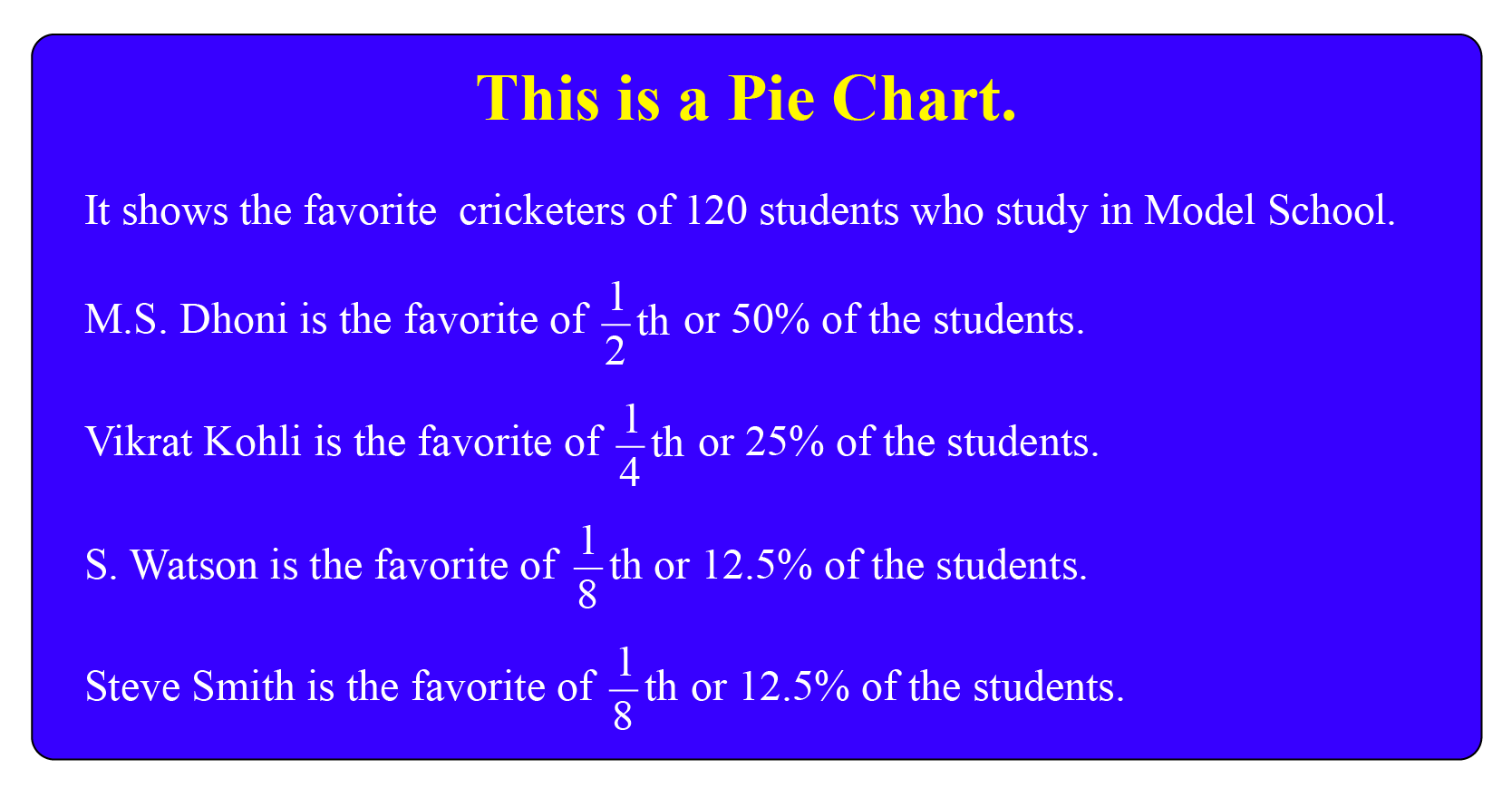### Bar-graph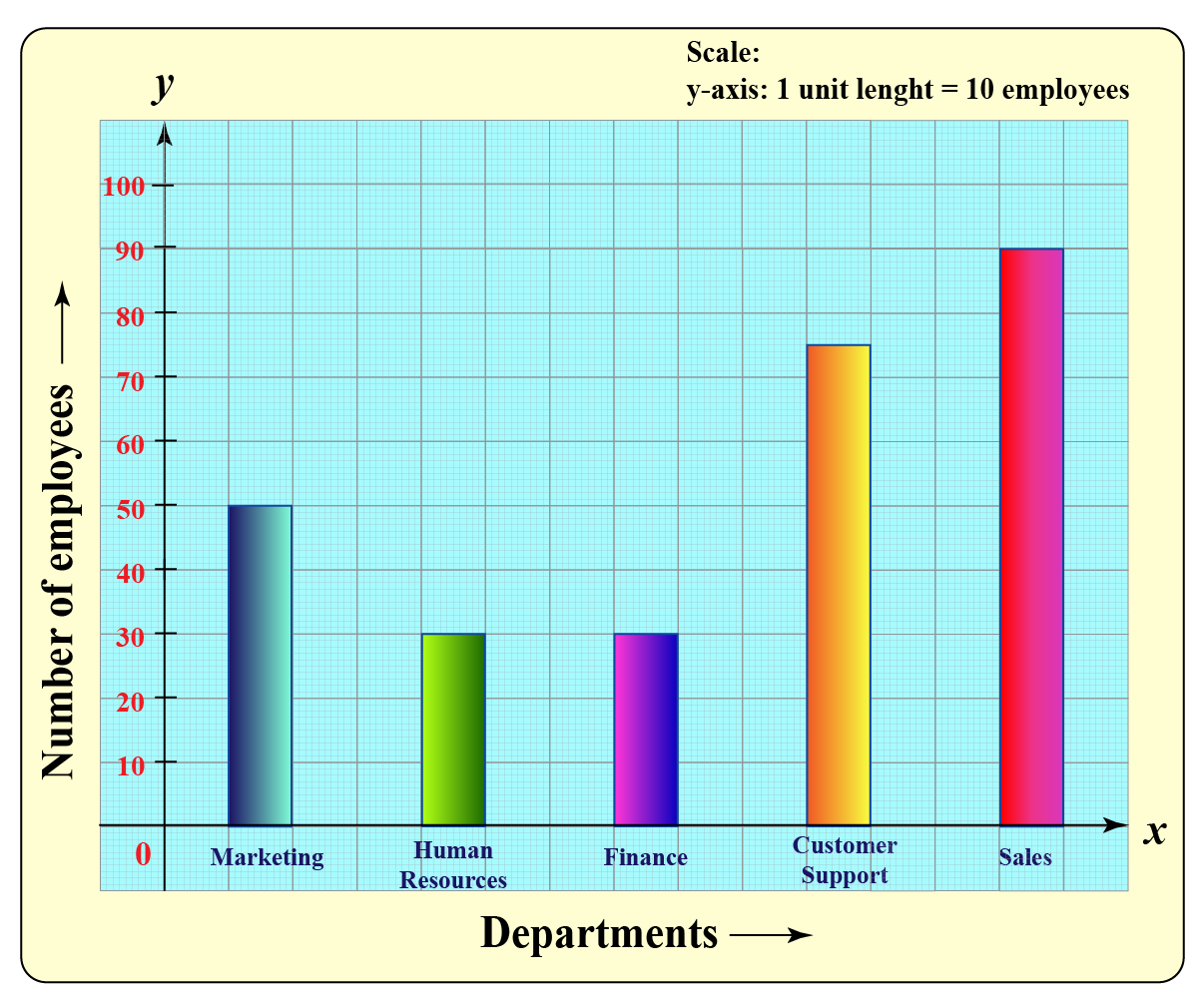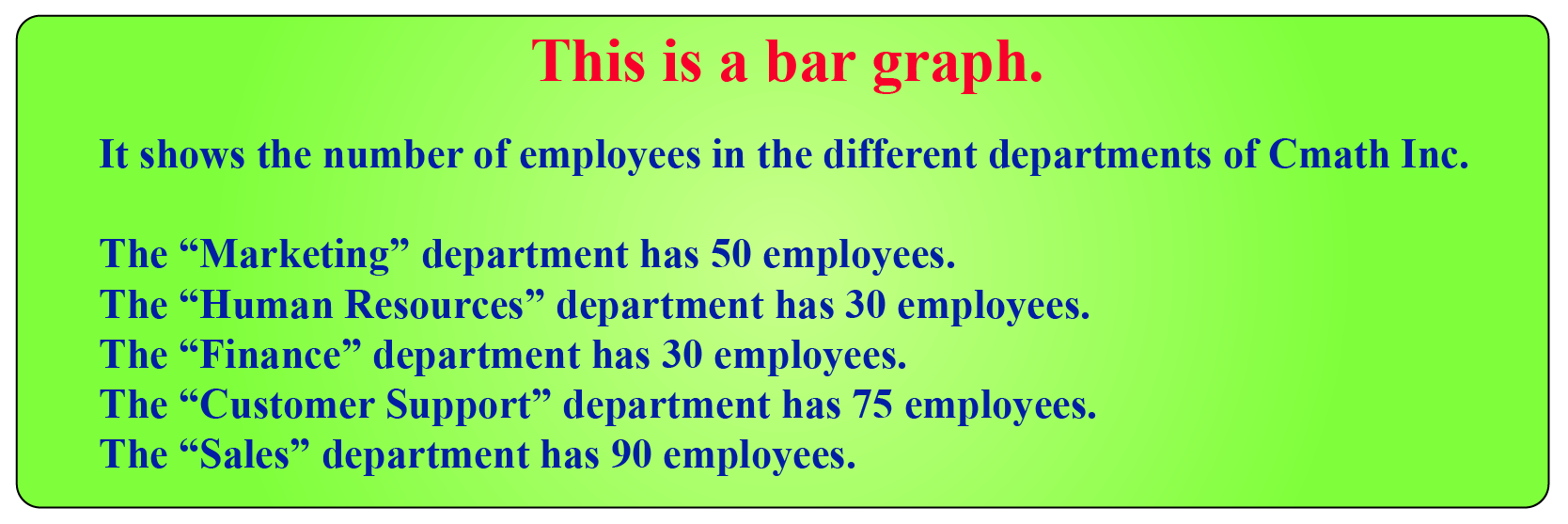### Line-graph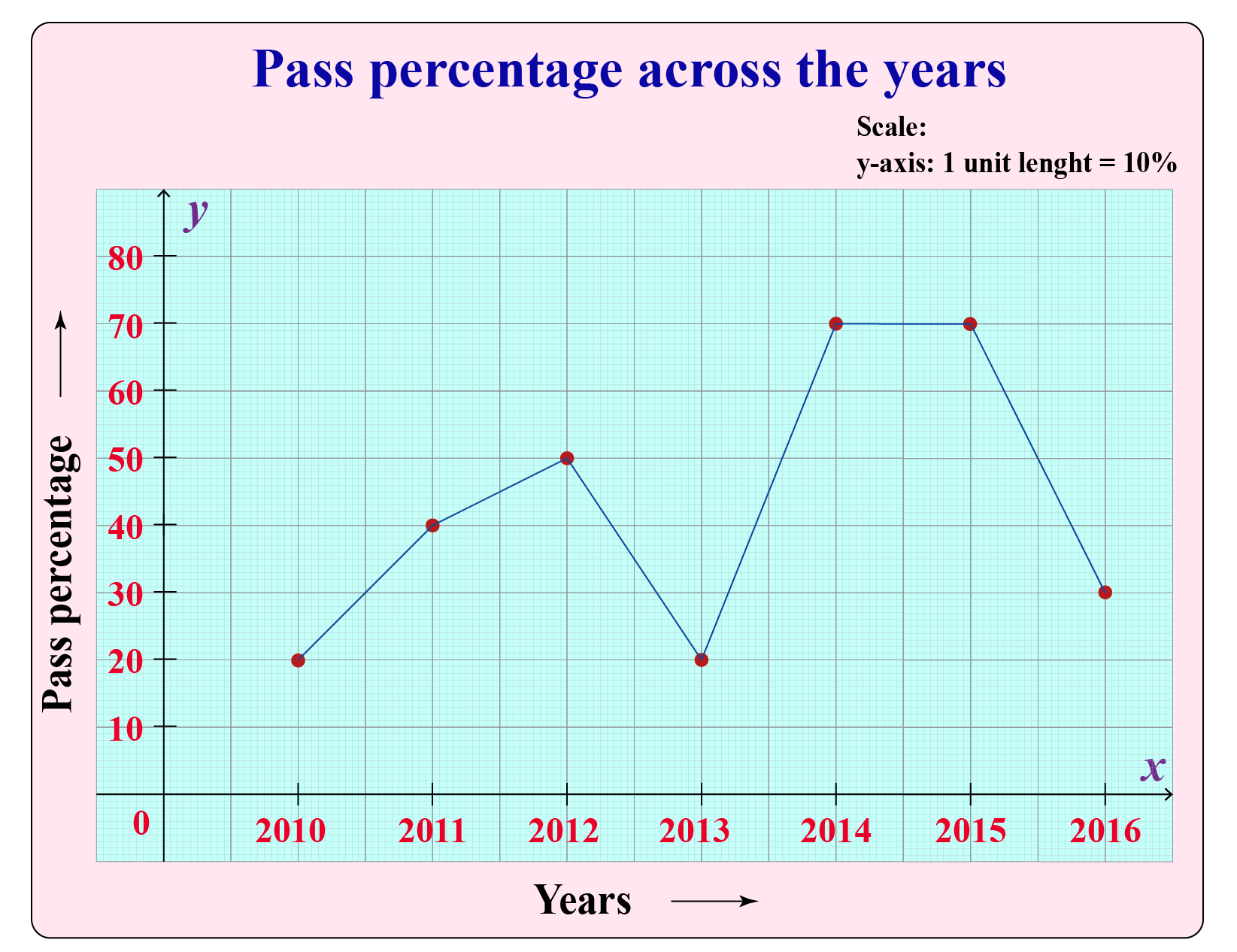### Histogram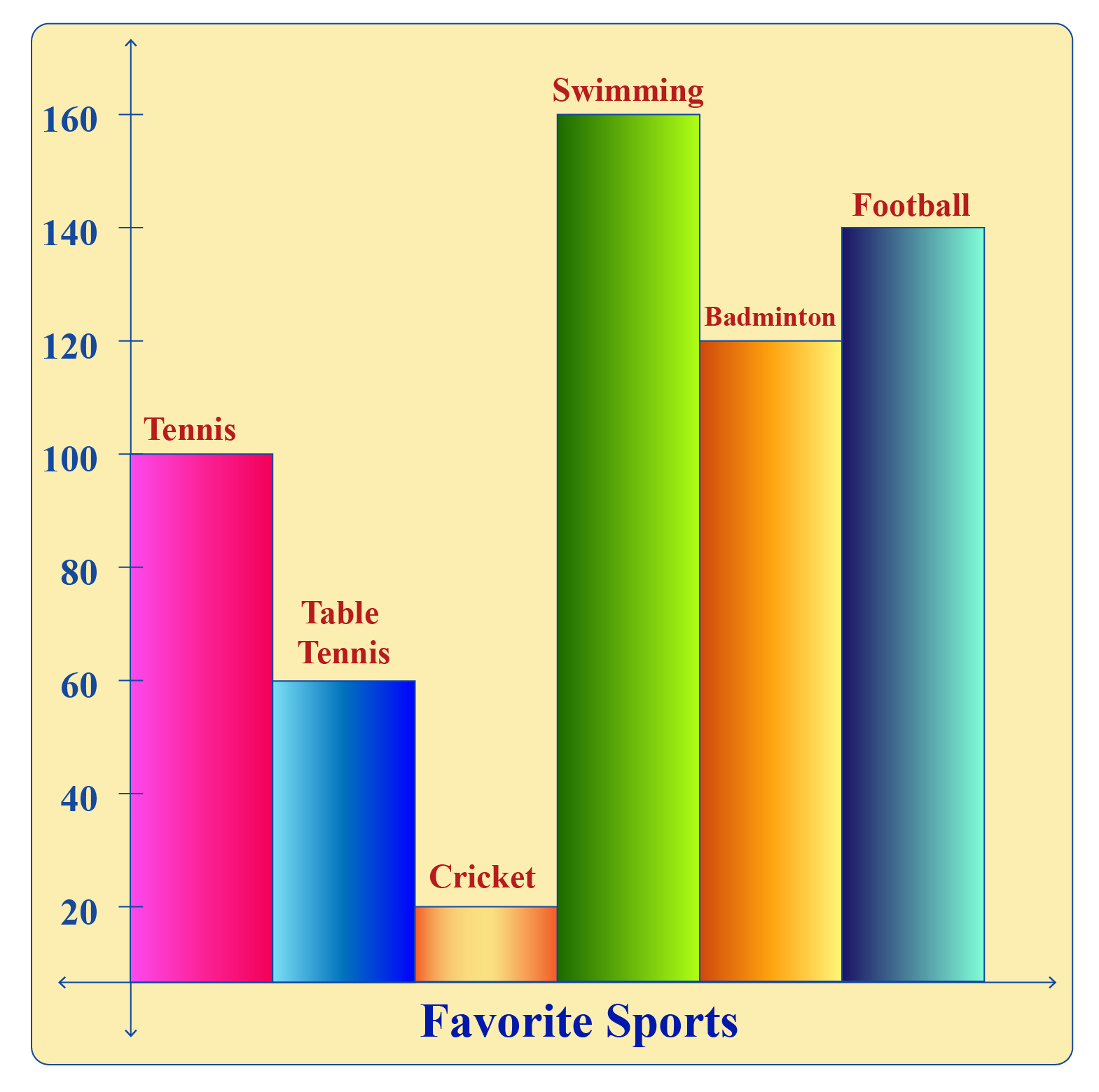## What Are The Different Types of Data

Talking about types of data, there are mainly two types of data: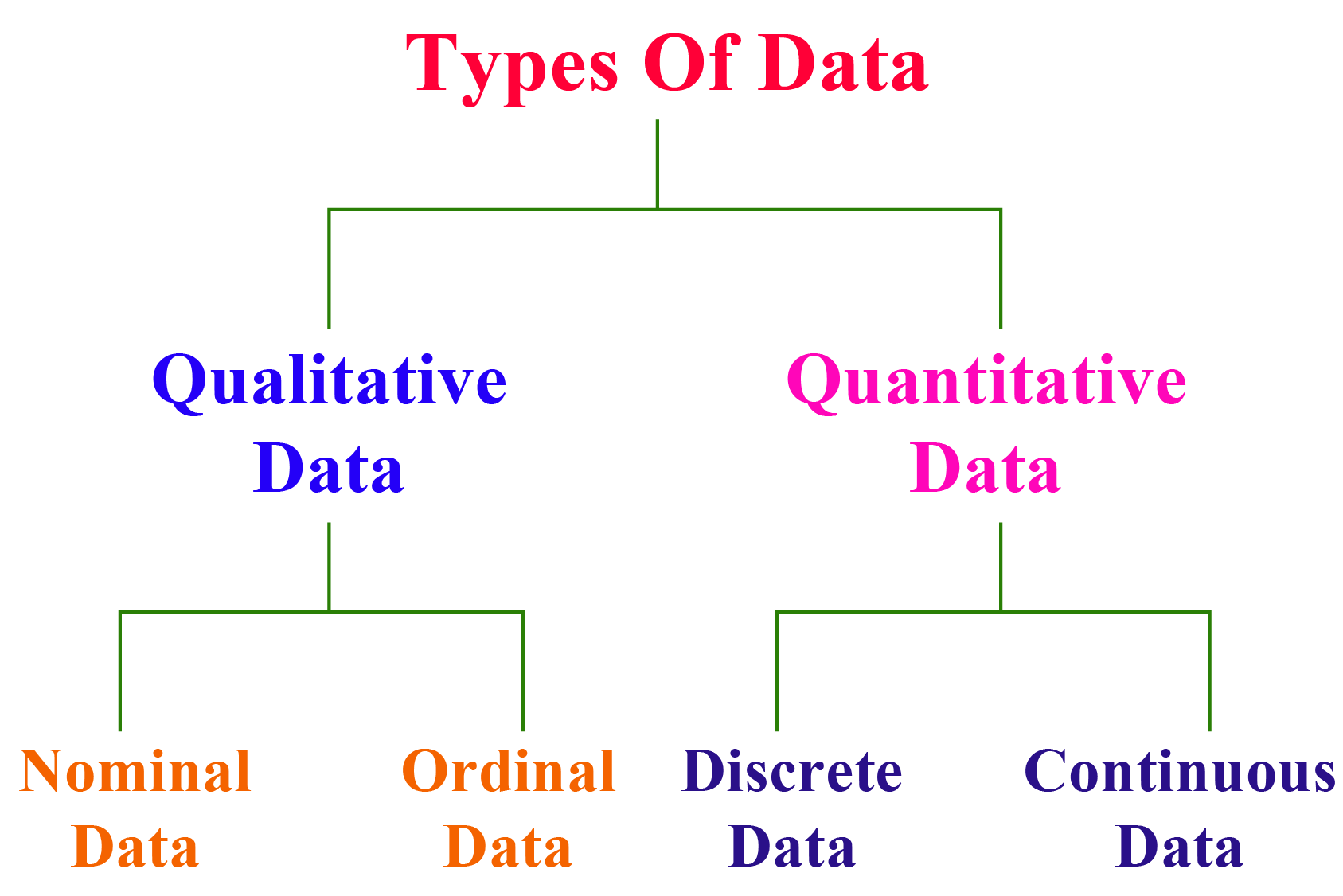• #### Qualitative Data - As the name itself suggests quality and quality means having uniqueness. Qualitative data is a type of data that can be observed and recorded. For example Gender, phone numbers, citizenship, etc.

Qualitative Data is further classified as:

• Nominal Data - It is a type of data mostly used for naming something or labeling or in classification. It is also called "named data". For example gender, country, race, eye color, hair color, hairstyle, etc.

An example is shown in the form of an image given below: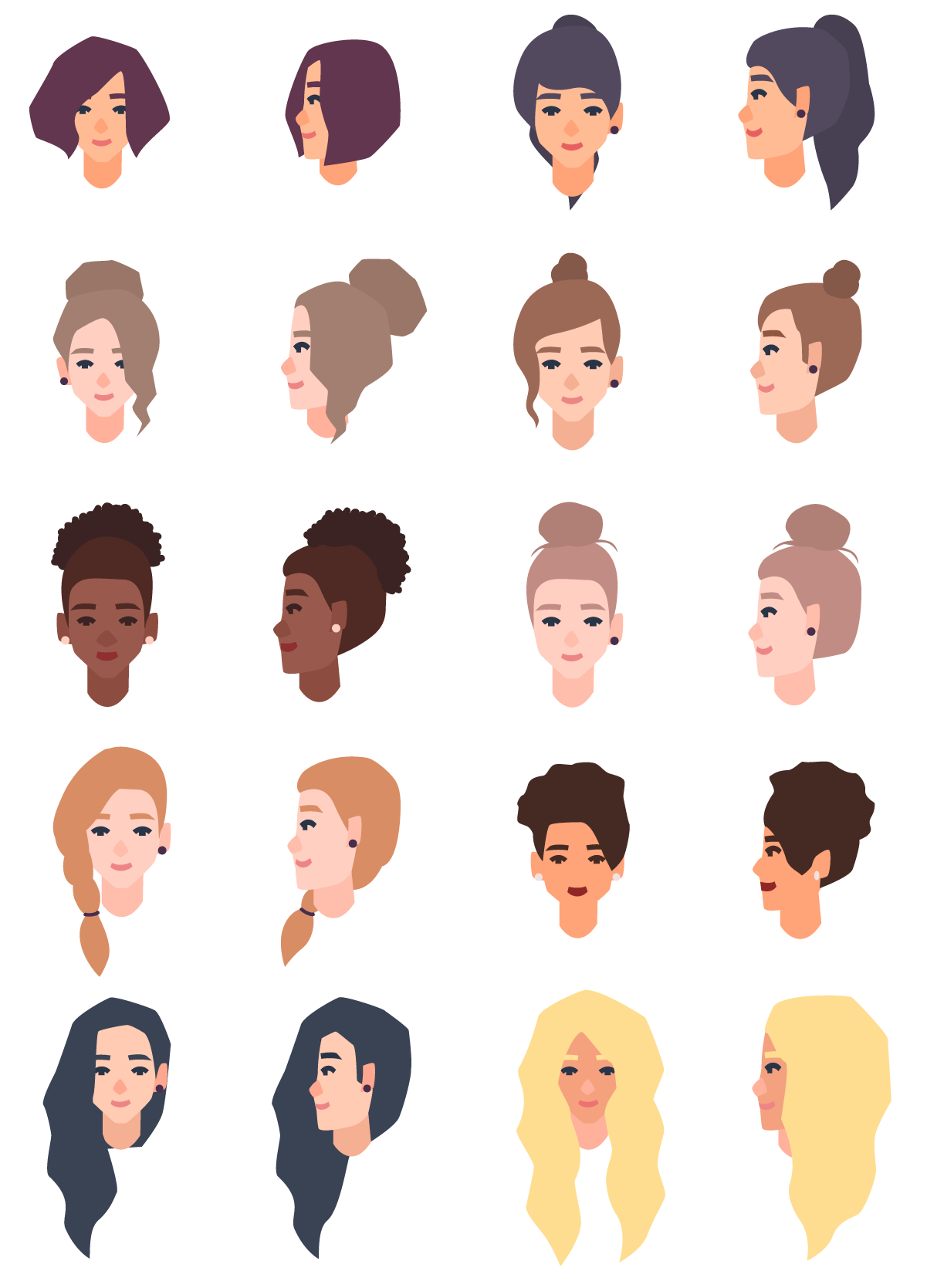• Ordinal Data -  It is a type of data that is named, ordered both, and a scale(range) is used. For example ranking in the class First, Second, Third.

An example is shown in the form of an image given below:• #### Quantitative Data - As the name suggests it deals with quantity and quantity is related to numbers. It is also known as numeric data. For example the number of bananas in a dozen, the number of candies in a box, etc.

Quantitative Data is further classified as:

• #### Discrete Data - This type of data takes values that can be counted such as counting the number of fruits on a tree, the number of students in a class, etc.

An example is shown in the form of an image given below: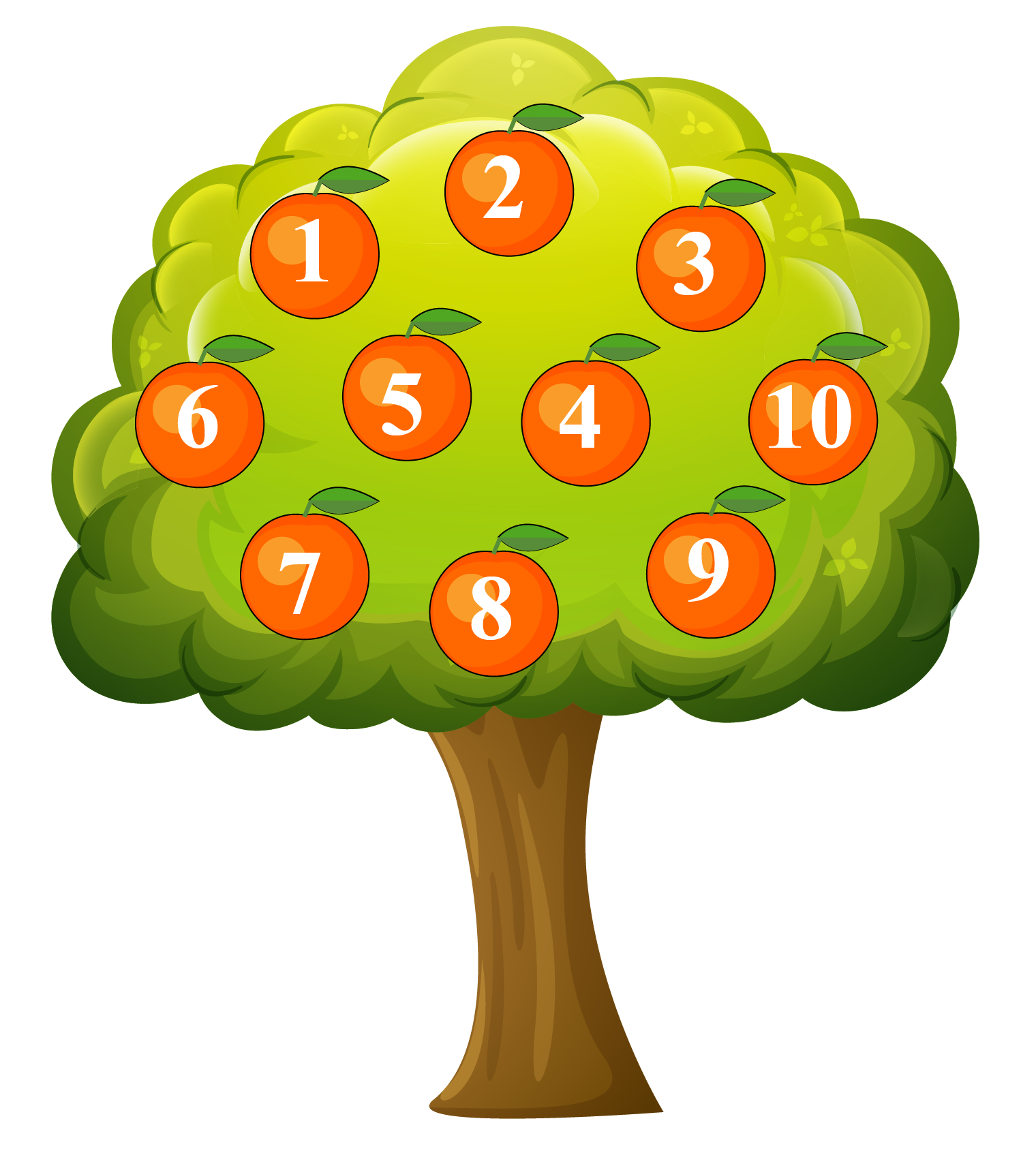• Continuous Data - This type of data takes specific values that can be measured and fall under a specific range such as weight, length, temperature, speed, etc.

An example is shown in the form of an image given below: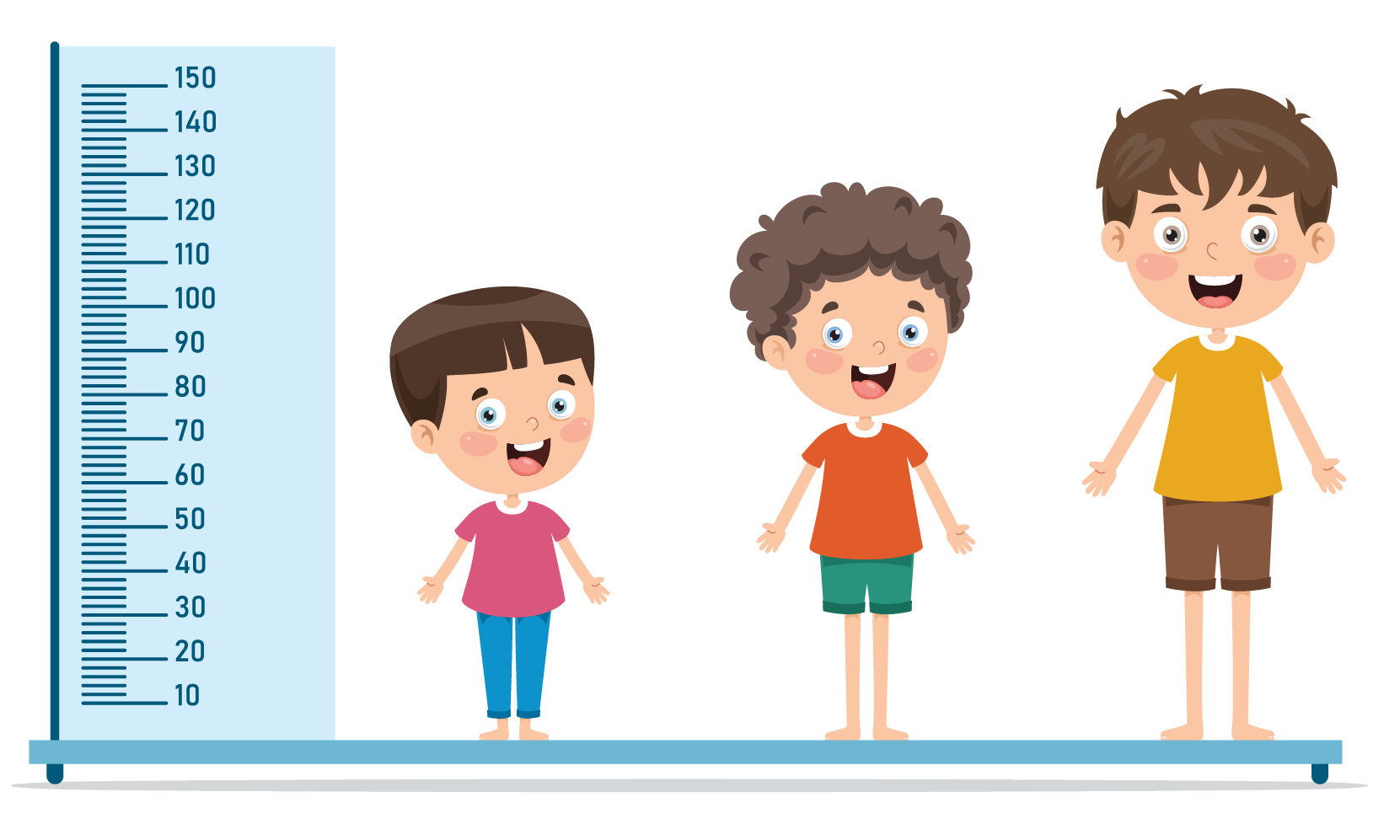Use this interesting simulation:

## Explain Primary And Secondary Data

Primary Data is a type of data that is gathered by the individual.

For example, The teacher giving an oral test and writing the marks.

More examples are letters, records, autobiographies, etc.Secondary data is a type of data that is gathered by someone else and used somewhere else.

For example, Another teacher using the marks obtained in an oral test for evaluation.

More examples: newspapers, encyclopedias, biographies, etc.Check out the interesting simulation given below: